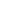# Free Â Class 10 Situational problems based on quadratic equations. Worksheets

Download free printable Â Class 10 Situational problems based on quadratic equations. Worksheets to practice. With thousands of questions available, you can generate as many Â Class 10 Situational problems based on quadratic equations. Worksheets as you want.

## Sample Â Class 10 Situational problems based on quadratic equations. Worksheet Questions

1.
 A train running at the speed of 60 km/hr crosses a pole in 9 seconds. What is the length of the train?
1.

120 metres

2.

180 metres

3.

324 metres

4.

150 metres

2.

If the price of a book is reduced by Rs. 5, a person can buy 5 more books for Rs. 300. Find the original list price of the book.

3.

The product of Taniya's age 5 years ago and his age 10 years later is 16. Determine Taniya present age.

4.

The perimeter of a rectangular field is 82 m and its area is 400 sq. m. Find the length and breadth of the rectangle.

5.

The length of the sides forming right angle of a right triangle are 5x cm and (3x-1). If the area of the triangle is . Find the hypotenuse.

6.

A train takes 2 hrs less for a journey of 300 km if speed is increased by 5 km/hr from its usual speed. Find the speed of train.

7.

The sum of squares of two consecutive odd number is 394. Find the numbers.

8.

The sum of squares of two consecutive even number is 340. Find the numbers.

9.

The difference of two numbers is 5 and the difference of their reciprocal is 1/10. Find the numbers.

10.

The sum of squares of two consecutive multiples of 7 is 637. Find the multiples.

Worksheets by UrbanPro

Our worksheets are designed to help students explore various topics, practice skills and enrich their subject knowledge, to improve their academic performance. Designed by Experts who have extensive experience and expertise in teaching a subject, these worksheets will improve your child's problem-solving skills and subject knowledge in a fun and interactive manner.
Check out our free customized worksheets across school boards, grades, subjects and levels of subject knowledge. You can download, print and share these worksheets with anyone, anywhere, anytime!

Get a custom worksheet to practice!

Select your topic & see the magic.

subjectSelect Chapter(s)

Chapters & Subtopics